# Working With Retail Purchases & Taxes

in Mathematics13 days ago

Hi there. In this educational math post, I go over the basic mathematics when it comes to comparing products with taxes and discounts in mind. This topic is very useful when it comes to purchasing decisions.

The motivation behind this post is when I was shopping around for new parts for a new computer build and buying a new computer monitor.Pixabay Image Source

## Topics

• Review Of Percents
• Calculating Final Prices After Taxes & Discounts
• Comparing Different Product Prices With Different Discounts

## Review Of Percents

Before we get right into calculating final prices, it is important to go over percents. Percents represent a portion or an amount of something. We can relate percents with fractions and decimals for calculations purposes.

One percent represents one hundredth or 0.01 of something. One percent is also 1 for each 100. This means that 1% of 100 is equal to 1. Taking 1% of 500 would be 5 as there are 5 hundreds in 500.

Example

Taking two percent of 200 would be 4. This is from doing 2 times 2 as there are two hundreds and we take 2 for each 100.

Example Two

A 10% tax on a \$500 TV purchase would result in an added \$50 tax.Pixabay Image Source

## Calculating Final Prices After Taxes & Discounts

In a previous mathematics post, I covered how to calculate final prices after sales discounts and taxes. The general formula looks like this: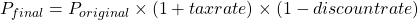Example One - Add 10% Tax

A computer graphics card costs \$1000. The sales tax for the region is 10%. What is the after tax price?

Convert the 10\$ tax into the 0.1 decimal form and apply the formula accordingly.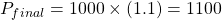The after tax price of this graphics card is \$1100.

Example Two - 10% Tax With A 10% Discount

Consider a pair of jeans that costs \$200. There is a sales tax of 10% with a 10% sales discount. What is the final price for the pair of jeans? (Hint: The answer is not \$200)

The formula setup would be the following: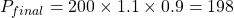The final price after the 10% discount and with the 10% tax for the pair of jeans is \$198.

Example Three - 13% Tax With A 15% Discount

A 32 inch curved screen computer monitor normally costs \$499. There is a 15% discount with a 13% tax. What is the final price for this computer monitor?

We have: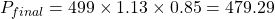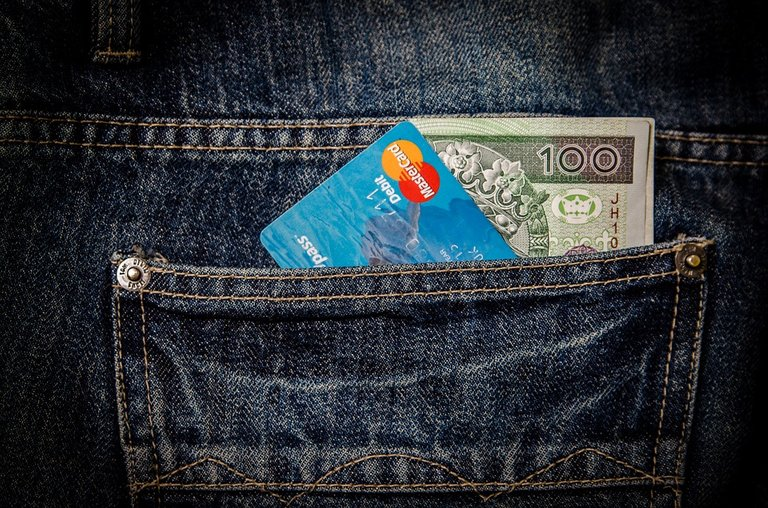Pixabay Image Source

## Comparing Different Product Prices With Different Discounts

A natural extension is comparing different product prices with different discounts. For simplicity, the tax rates are the same. Let's take a look at some examples.

Example One - CPU Chip A vs CPU Chip B

The first computer chip option costs \$220 while a second computer chip that is more powerful has a price of \$379. Assume a tax rate of 8%. What is the after-tax price difference between the two computer chip options?

The after tax price of the CPU chip option is: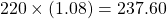For the second computer chip the after tax price is: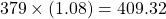The after tax price difference is \$409.32 minus \$237.60 which is equal to \$171.32. The price difference before tax was \$159 (379 - 220).

Example Two - Graphics Card 1 or Graphics Card 2

There are two graphics cards to choose from. Graphics Card 1 has a price of \$600 no discount. The second graphics card option that is more powerful costs \$1000 with a 7% discount. Assume a tax rate of 10%. What is the final price difference between the two graphics card options?

Graphics Card 1 has a final price of: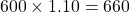The second graphics card has a final price of: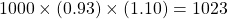Now that the final prices are found, we can compute the final price difference. This difference is \$1023 - \$660 = \$363.Pixabay Image Source